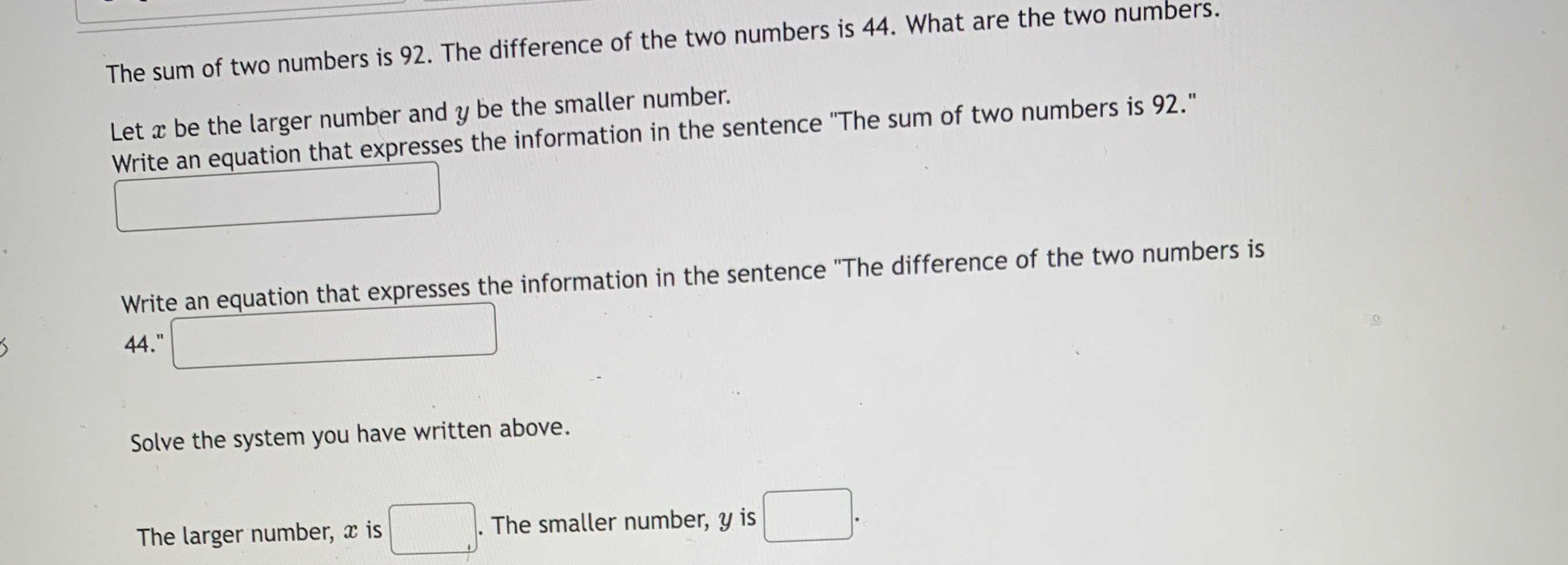### Still have math questions?

Algebra
QuestionThe sum of two numbers is $$92$$ . The difference of the two numbers is $$44$$ . What are the two numbers. Let $$x$$ be the larger number and $$y$$ be the smaller number. Write an equation that expresses the information in the sentence "The sum of two numbers is $$92$$ ."

Write an equation that expresses the information in the sentence "The difference of the two numbers is 44.""

Solve the system you have written above.

The larger number, $$x$$ is $$\square$$ . The smaller number, $$y$$ is $$\square$$

The sum of two numbers is 92.                   $$x+ y = 92$$
The difference of two numbers is 44.          $$x- y = 44$$
Adding these two equations, we get           $$2x = 136 ~~\Rightarrow~~ x = 68$$
Subtracting these two equations to get       $$2y = 48 ~~\Rightarrow~~ y = 24$$